NEET  >  NCERT Based Test: Torque on Current Loop & Magnetic Dipole

# NCERT Based Test: Torque on Current Loop & Magnetic Dipole

Test Description

## 15 Questions MCQ Test Physics Class 12 | NCERT Based Test: Torque on Current Loop & Magnetic Dipole

NCERT Based Test: Torque on Current Loop & Magnetic Dipole for NEET 2023 is part of Physics Class 12 preparation. The NCERT Based Test: Torque on Current Loop & Magnetic Dipole questions and answers have been prepared according to the NEET exam syllabus.The NCERT Based Test: Torque on Current Loop & Magnetic Dipole MCQs are made for NEET 2023 Exam. Find important definitions, questions, notes, meanings, examples, exercises, MCQs and online tests for NCERT Based Test: Torque on Current Loop & Magnetic Dipole below.
Solutions of NCERT Based Test: Torque on Current Loop & Magnetic Dipole questions in English are available as part of our Physics Class 12 for NEET & NCERT Based Test: Torque on Current Loop & Magnetic Dipole solutions in Hindi for Physics Class 12 course. Download more important topics, notes, lectures and mock test series for NEET Exam by signing up for free. Attempt NCERT Based Test: Torque on Current Loop & Magnetic Dipole | 15 questions in 15 minutes | Mock test for NEET preparation | Free important questions MCQ to study Physics Class 12 for NEET Exam | Download free PDF with solutions
 1 Crore+ students have signed up on EduRev. Have you?
NCERT Based Test: Torque on Current Loop & Magnetic Dipole - Question 1

### The magnetic moment of a current I carrying circular coil of radius r and number of turns N varies as

Detailed Solution for NCERT Based Test: Torque on Current Loop & Magnetic Dipole - Question 1

Magnetic Moment, M = NIA = NIπr2
i.e. M ∝ r2

NCERT Based Test: Torque on Current Loop & Magnetic Dipole - Question 2

### A 200 turn closely wound circular coil of radius 15 cm carries a current of 4 A. The magnetic moment of this coil is:

Detailed Solution for NCERT Based Test: Torque on Current Loop & Magnetic Dipole - Question 2

The magnetic moment is given by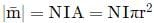= 200 × 4 × 3.14 × (15 × 10−2)2
= 200 × 4 × 3.14 × 15 × 15 × 10-4
= 56.5 A m2

NCERT Based Test: Torque on Current Loop & Magnetic Dipole - Question 3

### A uniform conducting wire of length length 10a and resistance R is wound up into four turn as a current carrying coil in the shape of equilateral triangle of side a. If current I is flowing through the coil then the magnetic moment of the coil is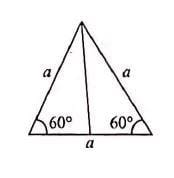Detailed Solution for NCERT Based Test: Torque on Current Loop & Magnetic Dipole - Question 3

Magnetic moment M = NIA
= 4 × I ×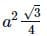= √3a2

NCERT Based Test: Torque on Current Loop & Magnetic Dipole - Question 4

The magnetic moment associated with a circular coil of 35 turns and radius 25cm, if it carries a current of 11A is

Detailed Solution for NCERT Based Test: Torque on Current Loop & Magnetic Dipole - Question 4

Given N = 35,
r = 25cm
= 25 × 10−2m
I = 11A
Then magnetic moment associated with this circular coil
M = NIA = NIπr2 = 35 × 11 × 3.14 × (25 × 10−2)2
= 75.56 A m2

NCERT Based Test: Torque on Current Loop & Magnetic Dipole - Question 5

A uniform conducting wire of length 18a and resistance R is wound up as current carrying coil in the shape of a regular hexagon of sides a. If the coil is connected to a voltage source V0, then the magnetic moment of coil is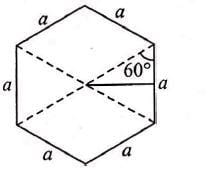Detailed Solution for NCERT Based Test: Torque on Current Loop & Magnetic Dipole - Question 5

In the regular hexagon, if each arm length is a,
then the number of turns in the given shape, n = 18a / 6a = 3
Now the area of given shape is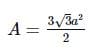Now magnetic moment M = nIA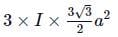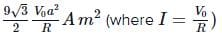NCERT Based Test: Torque on Current Loop & Magnetic Dipole - Question 6

The magnetic field at the centre of a circular loop of area A is B. The magnetic moment of the loop is

Detailed Solution for NCERT Based Test: Torque on Current Loop & Magnetic Dipole - Question 6

Let r be the radius of the circular loopMagnetic field at the centre of the loop isNCERT Based Test: Torque on Current Loop & Magnetic Dipole - Question 7

A short bar magnet has a magnetic moment of 0.65JT−1, then the magnitude and direction of the magnetic field produced by the magnet at a distance 8cm from the centre of magnet on the axis is

Detailed Solution for NCERT Based Test: Torque on Current Loop & Magnetic Dipole - Question 7

Here, M = 0.65JT−1,
d = 8cm
= 0.08m
The field produced by magnet at axial point is given by
B =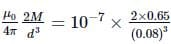= 2.5 × 10−4T along SN

NCERT Based Test: Torque on Current Loop & Magnetic Dipole - Question 8

A  circular coil of radius 10cm having 100 turns carries a current of 3.2A. The magnetic field at the center of the coil is

Detailed Solution for NCERT Based Test: Torque on Current Loop & Magnetic Dipole - Question 8

As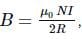Here N = 100,
I = 3.2A,
R = 10cm = 10 × 10−2m
∴ B =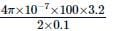= 2.01 × 10−3T

NCERT Based Test: Torque on Current Loop & Magnetic Dipole - Question 9

A circular coil of radius 10cm having 100 turns carries a current of 3.2A. For the coil given the magentic moment is

Detailed Solution for NCERT Based Test: Torque on Current Loop & Magnetic Dipole - Question 9

The magentic moment is given by
m = NIA = NIπr2 = 100 x 3.2 x 3.14 x (10 x 10-2)2
= 100 x 3.2 x 3.14 x 10-2
= 10 A m2

NCERT Based Test: Torque on Current Loop & Magnetic Dipole - Question 10

A circular coil of radius 10cm having 100 turns carries a current of 3.2A. the given coil is placed in a vertical plane and is free to rotate about a horizontal axis which coincides with its diameter. A uniform magnetic field of 5T in the horizontal direction exists such that initially the axis of the coil is in direction of the field. The coil rotates through an angle of 60∘ under the influence of magnetic field. The magnitude of torque on the coil in the final position is

Detailed Solution for NCERT Based Test: Torque on Current Loop & Magnetic Dipole - Question 10

Torque,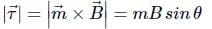Here, m = 10Am2, B=5T
Now initially θ = 0
Thus, initial torque, τi =0
In final position θ = 60
∴ τf = mBsin60∘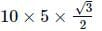= 25√3 N m

NCERT Based Test: Torque on Current Loop & Magnetic Dipole - Question 11

A current carrying loop is placed in a uniform magnetic field. The torque acting on it does not depend upon

Detailed Solution for NCERT Based Test: Torque on Current Loop & Magnetic Dipole - Question 11

The torque depends upon n, I, A, B and θ. But it does not depend upon the shape of the loop (rectangular, circular, triangular, etc.)

NCERT Based Test: Torque on Current Loop & Magnetic Dipole - Question 12

A circular coil of 70 turns and radius 5 cm carrying a current of 8 A is suspended vertically in a uniform horizontal magnetic field of magnitude 1.5 T. The field lines make an angle of 30o with the normal of the coil then the magnitude of the counter torque that must be applied to prevent the coil from turning is

Detailed Solution for NCERT Based Test: Torque on Current Loop & Magnetic Dipole - Question 12

N = 70, r = 5cm = 5 × 10−2m,I = 8A
B = 1.5T, θ = 30
The counter torque to prevent the coil from turning will be equal and opposite to the torque acting on the coil,
∴ τ = NIABsinθ = NIπr2Bsin30
= 70 × 8 × 3.14 × (5 × 10−2)2 × 1.5 × 1/2
= 3.297 N m
≈ 3.3 N m

NCERT Based Test: Torque on Current Loop & Magnetic Dipole - Question 13

A circular coil of 25 turns and radius 12 cm is placed in a uniform magnetic field of 0.5 T normal to the plane ol the coil. If the current in the coil is 6 A then total torque acting on the coil is

Detailed Solution for NCERT Based Test: Torque on Current Loop & Magnetic Dipole - Question 13

The torque acting on the coil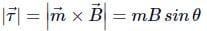Here the circular coil is placed normal to the direction of magnetic field then the angle between the direction of magnetic moment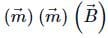is zero, then
τ = mBsinθ = mBsin0 = 0
∴ τ = 0

NCERT Based Test: Torque on Current Loop & Magnetic Dipole - Question 14

The final torque on a coil having magnetic moment 25 A m2 in a 5T uniform external magnetic field, if the coil rotates through an angle of 60 under the influence of the magnetic field is

Detailed Solution for NCERT Based Test: Torque on Current Loop & Magnetic Dipole - Question 14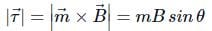Here, m = 25Am2; θ = 60; B = 5T
∴ τ = 25 × 5 × sin60 or
τ =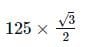= 108.25 N m

NCERT Based Test: Torque on Current Loop & Magnetic Dipole - Question 15

The magnitude of torque experienced by a square coil of side 12cm which consists of 25 turns and carries a current 10A suspended vertically and the normal to the plane of coil makes an angle of 30 with the direction of a uniform horizontal magnetic field of magnitude 0.9T is

Detailed Solution for NCERT Based Test: Torque on Current Loop & Magnetic Dipole - Question 15

τ = NIABsinθ
Here, N = 25,
I = 10A,
B = 0.9T,
θ = 30
A = a2 = 12 × 10−2 × 12 × 10−2
144 × 10−4m2
∴ τ = 25 × 10 × 144 × 10−4 × 0.9 × sin30 = 1.6 Nm

## Physics Class 12

157 videos|452 docs|213 tests
 Use Code STAYHOME200 and get INR 200 additional OFF Use Coupon Code
Information about NCERT Based Test: Torque on Current Loop & Magnetic Dipole Page
In this test you can find the Exam questions for NCERT Based Test: Torque on Current Loop & Magnetic Dipole solved & explained in the simplest way possible. Besides giving Questions and answers for NCERT Based Test: Torque on Current Loop & Magnetic Dipole, EduRev gives you an ample number of Online tests for practice

## Physics Class 12

157 videos|452 docs|213 tests

### How to Prepare for NEET

Read our guide to prepare for NEET which is created by Toppers & the best Teachers